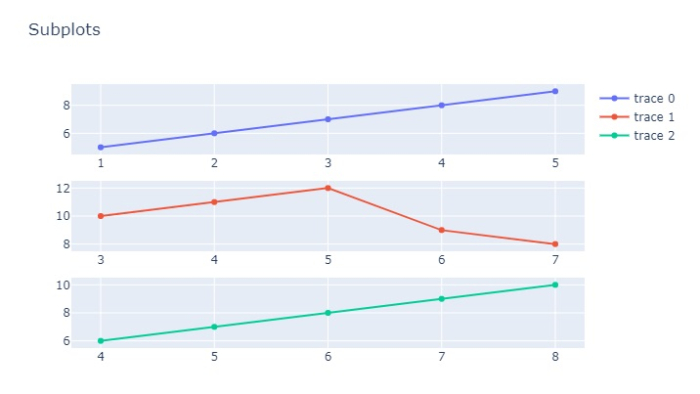# How to plot multiple figures as subplots in Python Plotly?

Plotly is an open-source Python library for creating charts. You can use the features available in Plotly to set multiple figures as subplots.

In this tutorial, we will use plotly.graph_objects to generate the figures. It contains a lot of methods to customize chart and render a chart into HTML format. For example, plotly.subplots() method can be used to add subplots.

Follow the steps given below to create subplots with Plotly express.

Step 1

Import plotly.graphs_objs module and alias as go.

import plotly.graphs_objs as go


Step 2

Import make_subplots to create subplots.

from plotly.subplots import make_subplots

Step 3

Create subplots for 3 rows and 1 column.

fig = make_subplots(rows=3, cols=1)

Step 4

Create the append_trace() method to append the Scatter plots.

fig.append_trace(go.Scatter(
x=[1,2,3,4,5],
y=[5,6,7,8,9],
), row=1, col=1)

fig.append_trace(go.Scatter(
x=[3,4,5,6,7],
y=[10,11,12,9,8],
), row=2, col=1)

fig.append_trace(go.Scatter(
x=[4,5,6,7,8],
y=[6,7,8,9,10]
), row=3, col=1)

Step 5

Use the update_layout() method to set the layout size.

fig.update_layout(height=400, width=400, title_text="Subplots")

## Example

Here is the complete code to plot multiple figures as subplots −

from plotly.subplots import make_subplots
import plotly.graph_objects as go

fig = make_subplots(rows=3, cols=1)

fig.append_trace(go.Scatter(
x=[1,2,3,4,5],
y=[5,6,7,8,9],
), row=1, col=1)

fig.append_trace(go.Scatter(
x=[3,4,5,6,7],
y=[10,11,12,9,8],
), row=2, col=1)

fig.append_trace(go.Scatter(
x=[4,5,6,7,8],
y=[6,7,8,9,10]
), row=3, col=1)

fig.update_layout(height=450, width=716, title_text="Subplots")
fig.show()

## Output

It will show the following output on the browser −